Select Page

# CBSE Vector Algebra Maths 12 Science Solutions for MCQ in English

CBSE Vector Algebra Maths 12 Science Solutions for MCQ in English to enable students to get Solutions in a narrative video format for the specific question.

Expert Teacher provides CBSE Vector Algebra Maths 12 Science Solutions for MCQ through Video Solutions in English language. This video solution will be useful for students to understand how to write an answer in exam in order to score more marks. This teacher uses a narrative style for a question from Vector Algebra not only to explain the proper method of answering question, but deriving right answer too.

Please find the question below and view the Solution in a narrative video format.

Question:

Solution Video in English:

You can select video Solutions from other languages also. Please check Solutions in ( Hindi )

## Similar Questions from CBSE, 12th Science, Maths, Vector Algebra

Question 1 : Find the area of the parallelogram whose adjacent sides are determined by the vectors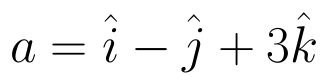and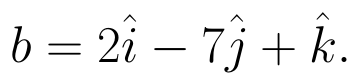(View Answer Video)

Question 2 : Find the scalar components of the vector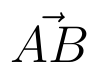with initial point A (2, 1) and terminal point B (-5, 7).  (View Answer Video)

Question 3 : Find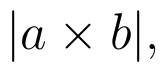if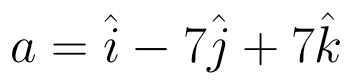and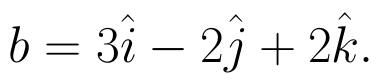(View Answer Video)

Question 4 : Find a unit vector in the direction of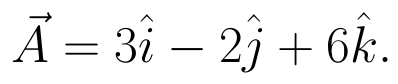(View Answer Video)

Question 5 : If the vertices A, B, C of a triangle ABC have position vectors (1, 2, 3), (-1, 0, 0), (0, 1, 2) respectively then find <ABC (<ABC is the angle between the vectors BA and BC). (View Answer Video)

### Linear Programming

Question 1 : The objective function is maximum or minimum, which lies on the boundary of the feasible region. (View Answer Video)

### Relations and Functions

Question 1 : Considergiven by f(x) = 4x + 3. Find the inverse of f. (View Answer Video)

Question 2 : A functiondefined as f(x) = 5x is, (View Answer Video)

Question 3 : Functions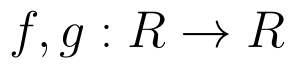are defined respectively, by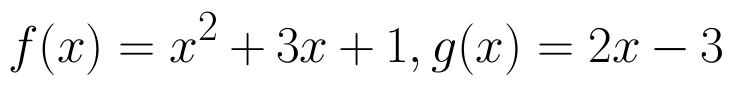, find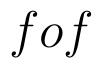(View Answer Video)

Question 4 : If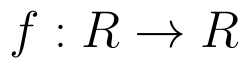is defined by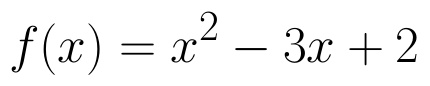, write f(f(x)). (View Answer Video)

Question 5 :  A function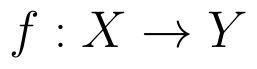is surjective if and only if , (View Answer Video)

### Continuity and Differentiability

Question 1 : Differentiate the function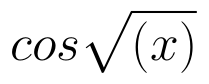with respect to x. (View Answer Video)

Question 2 : If x and y are connected parametrically by the equation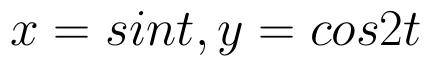, without eliminating the parameter, find. (View Answer Video)

Question 3 : Differentiate w.r.t.x the function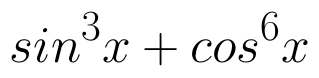. (View Answer Video)

Question 4 : Differentiate w.r.t.x the function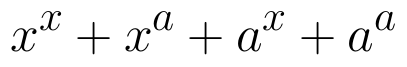, for some fixed a > 0 and x > 0. (View Answer Video)

Question 5 : If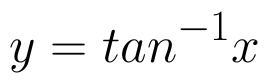, find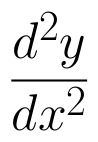in terms of y alone. (View Answer Video)